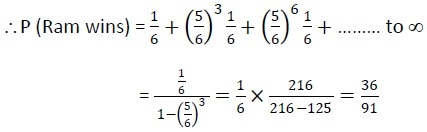# RRB NTPC 2021 Exam (Phase-1) Memory Based Maths Questions with Answers: Check Mathematics Questions asked in RRB NTPC 2020-21 CBT

RRB NTPC 2021 Exam (Phase-1) Memory Based Maths Questions with Answers: Get the memory based questions from Mathematics Section that came in RRB NTPC 2020 Exam held in online mode from 28th December 2020 till 13th Jan 2021.

Created On: Jan 13, 2021 11:54 ISTRRB NTPC 2021 Exam (Phase-1) Memory Based Maths Questions with Answers: Check Mathematics Questions asked in RRB NTPC 2020-21 CBT

RRB NTPC 2021 Exam (Phase-1) Memory Based Maths Questions with Answers: RRB NTPC 2020-21 Exam has been commenced from 28th December 2020. Today, the exam was conducted for the RRB Non-Technical Popular Categories (NTPC) 35208 Graduate & Under-Graduate Posts in two shifts. RRB NTPC 2020 Exam will be conducted for around 23 lakh Candidates in Phase-1, i.e., from 28th December 2020 to 13th January 2021 and for around 27 Lakh Candidates in Phase-2, i.e., from 16th January to 30th January 2021.

Check RRB NTPC 2020 Exam Analysis - Difficulty Level & Good Attempts

In this article we are going to share the important memory based General Intelligence & Mathematics section Questions as per the feedback received by the candidates who have appeared for RRB NTPC 2020 Online Exam. Candidates are advised to definitely cover these questions for scoring high marks in the Exam. Let’s have a look at the Important Questions that are being covered in the RRB NTPC 2020 Exam:

Check RRB NTPC 2020 Exam Schedule

## RRB NTPC 2021 Mathematics Section Memory Based Questions with Answer (Phase-1)

1. The marked price of an article for sale is 20% of its cost price. How much percent does the dealer gain by allowing a discount of 15%?

a) 5%

b) 7%

c) 10%

d) 6.25%

Solution: Let the CP of the article be Rs. 100

Marked Price = 100 ×100/80 = 𝑅𝑠. 125

Then, SP after discount = 125 × 85/100 = Rs. 106.25

Gain Percent = (106.25 − 100/100) × 100 = 6.25%

Check GA/GK/Current Affairs Memory Based Questions with Answers of RRB NTPC 2020 Exam

2. A sells an article to B on 20% profit. B sells back the article to A on 20% loss. In the whole transaction:

a) A left with no profit and no loss

b) A earns 20% profit

c) A earns 24% profit

d) B incurs 40% loss

Solution: Let the CP of A be Rs.100

Then, CP of B = 100 + 20% = Rs. 120

SP of B = 120 (100 − 20)/100 = 𝑅𝑠. 96

Total profit of A = (120 – 100) + (100 – 96) = 24%

Check RRB NTPC 2020 Memory Based General Science Questions - Biology/ Chemistry/ Physics

3. A and B together can complete a work in 10 days. A alone can complete the work in 15 days. If B does the work only half a day daily, then in how many days A and B together will complete the work?

a) 10 Days

b) 15 Days

c) 12 Days

d) 20 Days

Solution: B’s 1 day’s work = 1/10− 1/15 = 1/30

B’s 1/2 day’s work = 1/60

(A+B) together will complete work in = 1/15 + 1/60 = 1/12 = 12 days

Check RRB NTPC 2020-21 Memory Based Indian Polity Questions with Answers

4. A and B together can complete a piece of work in 20 days. B and C together can complete in 30 days. If A is twice as good a workman as C, then in how many days will B alone can complete the same work?

a) 30 Days

b) 60 Days

c) 40 Days

d) 10 Days

Solution: A+B’s 1 day’s work = 1/20

B+C’s 1 day’s work = 1/30

Since, A is twice as good a workman as C.

∴ A = 2C

B+2C = 1/20

C = 1/20 - 1/30 = 1/60

B can complete the same work = 1/30 - 1/60 = 1/60 = 60 Days

Check RRB NTPC 2020 GK History Memory Based Questions with Answers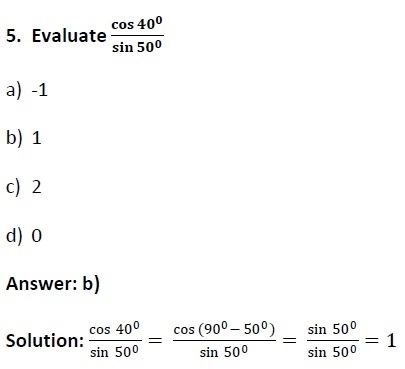Check RRB NTPC 2020 GK Geography Memory Based Questions with Answers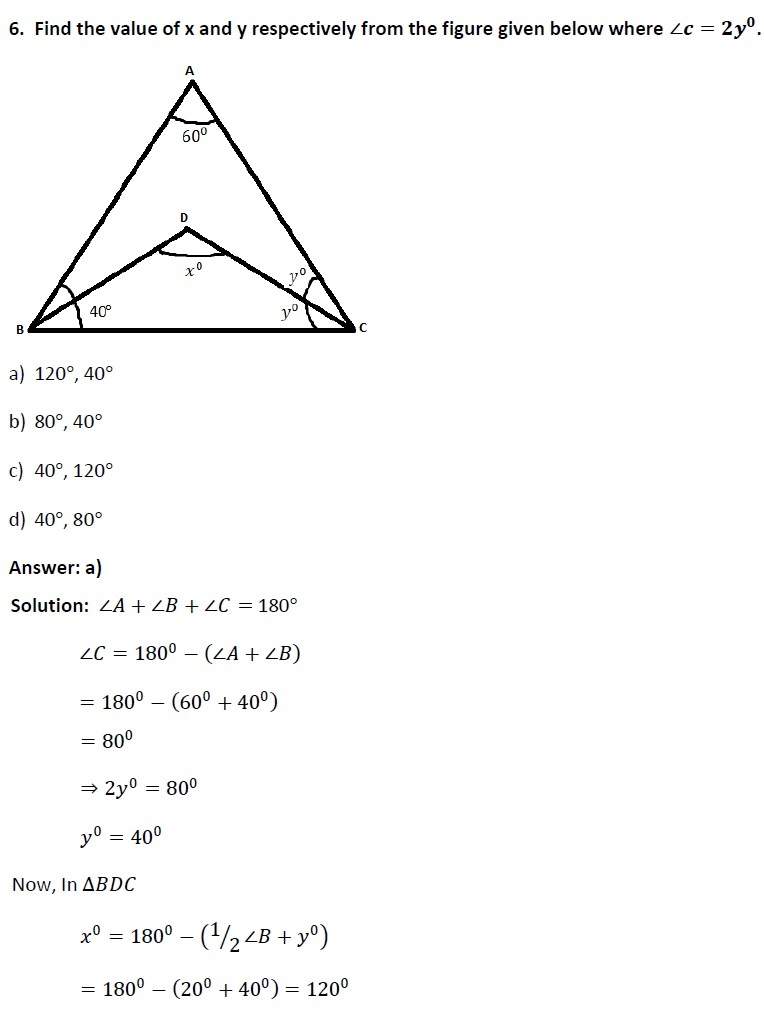Check RRB NTPC 2020 Memory Based Reasoning Questions with Answers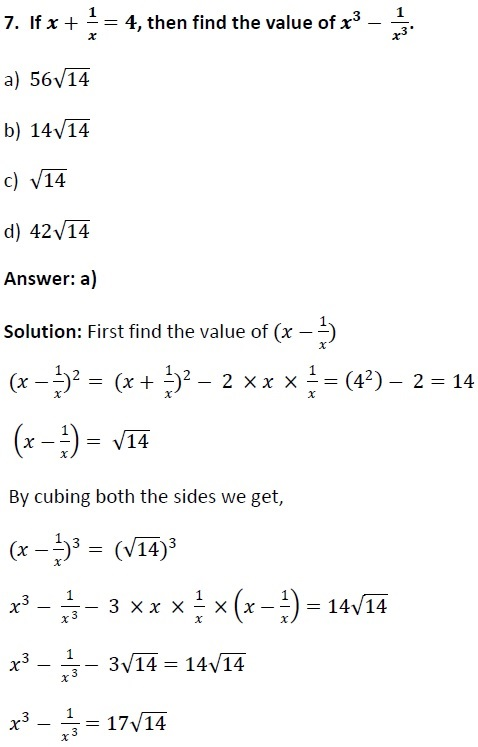8. What will be the simple interest on an amount of Rs. 2000 in 3 years at interest 4% per annum?

a) Rs. 280

b) Rs.240

c) Rs.250

d) Rs. 220

Solution: Simple Interest = 𝑃 ×𝑅 × 𝑇/100

2000 ×4 ×3/100 = Rs.240

Check RRB NTPC 2020 Exam 7 Last Minute Preparation Tips

9. If x−1/𝒙 = 3, then value of x3 − 1/𝒙𝟑 is:

a) 32

b) 36

c) 40

d) 49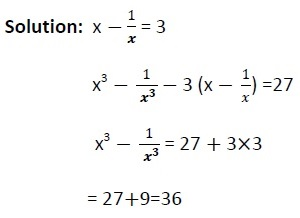10. If a +b +c = 6, a2+b2+c2=14 and a3+b3+c3 = 36, then value of abc is:

a) 3

b) 5

c) 6

d) 12

Solution:

a +b + c = 6

a2+b2+c2=14

a3+b3+c3 = 36

Put value as

A = 1, b = 2, c = 3

1 + 2 + 3 = 6

1+4+9=14

1+8+27=36

∴ abc = 1×2×3 = 6

Check Detailed RRB NTPC 2020 Exam Pattern & Syllabus

11. The average weight of 20 students in a class is 44 kg and that of the remaining 10 student is 38. Find the average weights of all the students the class:

a) 39 kg

b) 43 kg

c) 41 kg

d) 42 kg

Solution: Total weight of 20 student = 20 × 44 = 880

Total weight of 10 student = 10 × 38 = 380

Average weights of all the students = (880 + 380)/30 =1260/30 = 42 kg

Check RRB NTPC 2020 Eligibility Criteria

12. The difference in C.I and S.I for 2 years on a sum of money is Rs.320. If the S.I for 2 years be Rs.5760, the rate percent is:

a) 105/9%

b) 100/8%

c) 100/9%

d) 9%

Solution: S.I for 1 year = Rs.2880

S.I on Rs. 2880 for 1 year = Rs.320

Hence, rate percent = 320*100/2880 = 100/9%

Check RRB NTPC Salary after 7th Pay Commission, Job Profile and Promotion Policy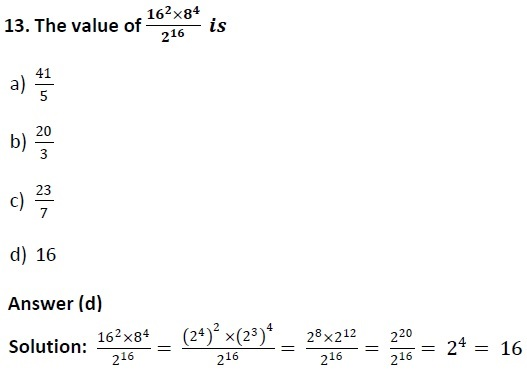Check RRB NTPC 2020 Exam & Admit Card Rules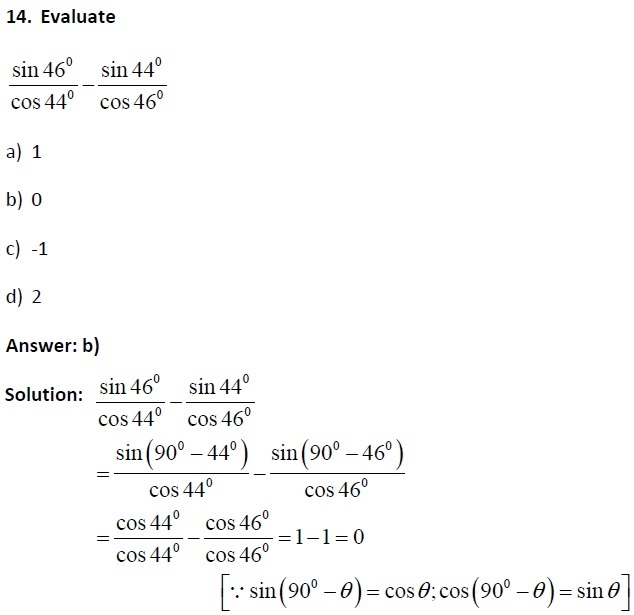Check the Minimum Qualifying Marks for RRB NTPC 2020 Exam

15. Two trains starting at the same time from two stations 400 km apart and going in opposite direction cross each other at a distance of 230 km from one of the stations. What is the ratio of their speeds?

a) 11:9

b) 23:17

c) 18:4

d) None of these

Solution: In same time, they cover 230 km and 170 km respectively.

For the same time speed and distance is inversely proportional.

So ratio of their speed = 230 : 170 = 23 : 17

16. If 𝒂 + 𝒃= 𝒄 , then the value of the expression 𝑎3+𝑏3 − 𝑐3 −𝟑𝒂𝒃𝒄 will be:

a) 0

b) 3abc

c) -3abc

d) 𝑐3

Solution: 𝑎+ 𝑏 = 𝑐

𝑎 + 𝑏 – 𝑐 = 0 ⇒ 𝑎 + 𝑏 + (−𝑐) = 0

⇒ 𝑎3+𝑏3+ (−𝑐3)+3𝑎𝑏 −𝑐 = 0

⇒ 𝑎3+𝑏3 − 𝑐3−3𝑎𝑏𝑐 = 0

Check RRB NTPC 2021 Books & Authors GK questions with Answers

Check RRB NTPC 2021 GK Important Days Memory Based Questions with Answers

Check RRB NTPC 2021 GK Sports & Awards Memory Based Questions with Answers

17. Ramesh, Mahesh and Suresh throw a dice in succession till one gets a ‘six’ and wins the game. Then the probability of Ramesh’s winning is:

a) 25/91

b) 36/91

c) 31/90

d) None of these